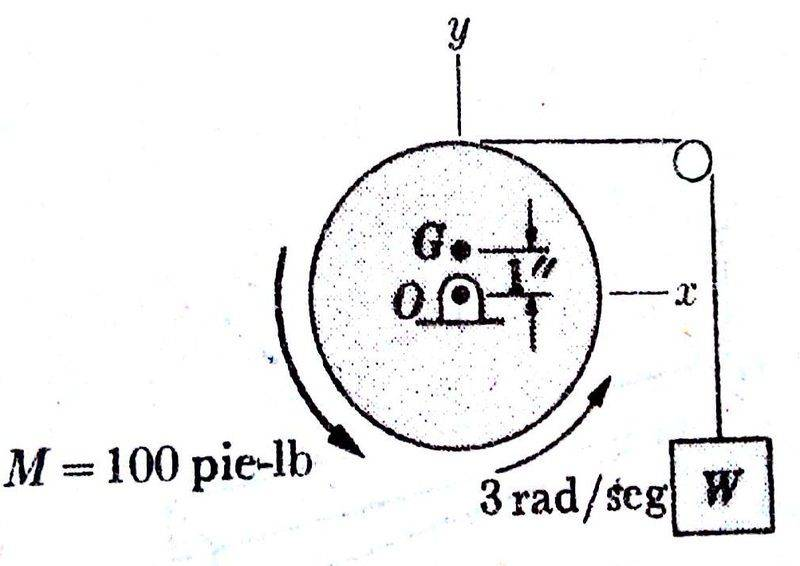# Rigid body

skxnxr
Member warned that homework questions must include an effort

## Homework StatementAn unbalanced pulley 8in. radio. and 32.3 pounds weighing. It has a radius of gyration of 6in with respect to its axis. When a pair M = 100pie.lb applies, lifts a weight W = 96.6 pounds. In the position shown in the figure, the pulley has an angular velocity of 3 rad / sec in counter-clockwise. Determining the tension in the string T and axial components of Ox and Oy, of the reaction in O. Neglect reaction friction and suppose the periphery of the small pulley is smooth. Thanks

Book results:
T=141.6 lb
Ox=-143.7 lb
Oy=31.45 lb

## Homework Equations

: (rigid body dynamics)[/B]
∑F=m.a
ΣT=(ri-R) x (mi ai)

## The Attempt at a Solution

I try to raise the equations of rigid body dynamics, but I'm nowhere near the result. I think I have to take into consideration the imbalance of the pulley. How should raise the equations? Thanks

## Answers and Replies

Mentor
I try to raise the equations of rigid body dynamics, but I'm nowhere near the result.
Whether they are correct or incorrect, please show us what you have tried.

skxnxr
I try this:
∑F=m.a
T - m.g = m.ay
T = m(ay + g)
T = 32,3 (ay + 32,2)
I need to calculate ay.

ΣT=IO.α
T.6in = IO.α
6T = (½ . m . r^2).α
6T=(½ . 32,3 . 6^2) . 3
T = 289. This result is not correct